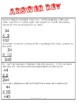# Two-Digit by One-digit Multiplication Story Problems: Multi StepSubject
Resource Type
File Type

PDF

(366 KB)
Product Rating
Standards
• Product Description
• StandardsNEW

This is a practice sheet for multiplication story problems that are for two-digit by one digit problems. There are also some two digit numbers that are multiplied by ten. These story problems have several steps, use a chart, have follow up questions and asks the student come up with their own story problem to solve at the end. It can be used as a whole group practice or as a practice in a math center. Answer key included!

Solve multistep word problems posed with whole numbers and having whole-number answers using the four operations, including problems in which remainders must be interpreted. Represent these problems using equations with a letter standing for the unknown quantity. Assess the reasonableness of answers using mental computation and estimation strategies including rounding.
Multiply or divide to solve word problems involving multiplicative comparison, e.g., by using drawings and equations with a symbol for the unknown number to represent the problem, distinguishing multiplicative comparison from additive comparison.
Solve two-step word problems using the four operations. Represent these problems using equations with a letter standing for the unknown quantity. Assess the reasonableness of answers using mental computation and estimation strategies including rounding.
Use multiplication and division within 100 to solve word problems in situations involving equal groups, arrays, and measurement quantities, e.g., by using drawings and equations with a symbol for the unknown number to represent the problem.
Total Pages
N/A
N/A
Teaching Duration
N/A
Report this Resource to TpT
Reported resources will be reviewed by our team. Report this resource to let us know if this resource violates TpT’s content guidelines.